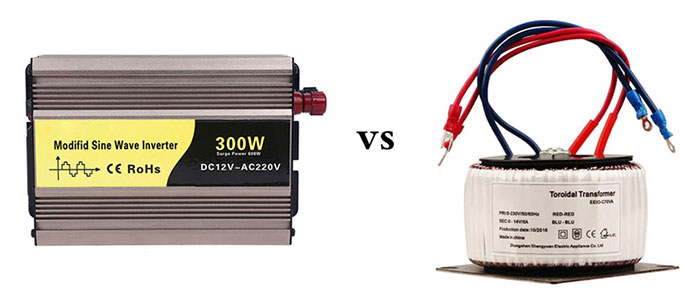# Difference between Inverter and Transformer

Inverter is commonly used in the industrial field and its main function is to convert the current in a certain way. Transformer is a device that can change the AC voltage based on the electromagnetic induction principle. It is mainly composed of primary coil, secondary coil and iron core (magnetic core). To make it easier to understand the power inverter, hereby the difference between inverter and transformer will be discussed.Difference between inverter and transformer

• Different uses

The inverter is a kind of electric device that can convert current from DC to AC. Actually it is the about the voltage inverting process. Normally, the 220V AC is transformed into DC, however, the inverter works in the opposite method. For example, a power inverter can convert the 12V DC voltage into the current with high frequency and high voltage.

The transformer is a device that can realize electric energy conversion based on the electromagnetic induction rule. It can convert the AC energy of certain voltage and current into another AC with certain voltage and current of the same frequency. It can improve the voltage of the power generated by the power station so as to reduce the loss in long-distance power transmission process. It can also gradually reduce the high voltage to the low voltage at the power application place so that users can use the power. It can be seen that transformer is very important for the power grid. It is the key device to ensure the safe, reliable and economic-saving operation of the power grid and to ensure the normal power supply for people’s production and living activities.

• Different classification

The power inverter can be classified based on the sinusoidal property, current source property, electric-grid network type, topology structure and power level. The transformer is classified based on the phase number, cooling mode, application, winding method and iron core.

• Different functions

The power inverter has five protection functions, separately are short circuit, overload, over-voltage or under-voltage and over-temperature protections. The main functions of the transformer include voltage transformation, current transformation, impedance transformation, isolation and voltage stabilization (magnetic saturation and transformer), etc.

Is it possible to use the transformer as the inverter?

Of course not. Inverter is an assembled inverting device and it is essentially different with the transformer. For the inverter, the input current is DC and the output current is AC. The work principle is same with that of the switch power supply. But its oscillation frequency is within a certain range. For example, if the frequency is 50HZ and then the frequency of the output AC is 50HZ. Therefore, the output frequency of the power inverter can be changed. The transformer is a device with specific frequency. The input and output currents are both AC. But its output voltage level can be adjusted. For instance, the input and output currents of the industrial frequency transformer (the common transformer) are both AC. And it can work only when the frequency is within 40-60HZ.